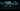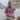# The Legend of JavaScript Equality Operator

Posted January 4, 2016Start discussion

Sometimes when I’m writing Javascript I want to throw up my hands and say ‘this is bullshit!’

During everyday JavaScript coding, it may be hard to see how the equality operator works. Especially when the operands have different types. Time to time this creates bugs in conditionals, which are difficult to identify. It is easy to understand why `0 == 8` is `false` or `'' == false` is `true`. But why `{} == true` is `false` is not obviously to see. If you’re interested:

• to know better the equality and identity operators
• to learn the formal computation algorithm
• to practice a plenty of examples

then make yourself comfortable and let’s begin.

The following terminology is used in the article:

• Operator is a symbol denoting an operation.
For example the equality operator `==` compares two values, identity operator `===` compares two values and their types, addition operator `+` sums two numbers or concatenates two strings.
• Operand is the subject of the operation, a quantity on which an operation is performed.
In the expression `0 == {}`, the `0` is the first operand and `{}` the second.
• Primitive types in JavaScript are considered numbers, strings, booleans, null and undefined.

## The identity operator

JavaScript is performing the equality evaluation. The interpreter first converts both operands to the same type. Then executes the identity comparison.
The identity evaluation algorithm (IEA) `===`:

1. If both operands have different types, they are not strictly equal
2. If both operands are `null`, they are strictly equal
3. If both operands are `undefined`, they are strictly equal
4. If one or both operands are `NaN`, they are not strictly equal
5. If both operands are `true` or both `false`, they are strictly equal
6. If both operands are numbers and have the same value, they are strictly equal
7. If both operands are strings and have the same value, they are strictly equal
8. If both operands have reference to the same object or function, they are strictly equal
9. In all other cases operands are not strictly equal.

The rules are quite simple.
It is worth mentioning that `NaN` in identity (and in equality) operator compared with any other value always evaluates to `false`. Lets consider some examples. It’s the best way to remember the strict comparison algorithm.

Example 1

``1 === "1" // false, IEA rule 1``

Operands are different types (number and string) and based on IEA rule 1 they are not identical.

Example 2

``0 === 0 // true, IEA rule 6``

Operands are the same type (number) and have the same value, so they are strictly equal based on IEA rule 6.

Example 3

``undefined === undefined // true, IEA rule 3``

Both operands are `undefined` and applying the IEA rule 3 it’s an equality.

Example 4

``undefined === null // false, IEA rule 1``

Because operands are different types, based on IEA rule 1 they’re not identical.

Example 5

``NaN === NaN // false, IEA rule 4``

Operands are the same type (numbers), but the IEA rule 4 indicates than nothing is equal with a `NaN`. The result is `false`.

Example 6

``````var firstObject = {},
secondObject = firstObject;
secondObject['name'] = 'Neo';
secondObject === firstObject // true, IEA rule 8``````

Both variables `firstObject` and `secondObject` are references to the same object and based on IEA rule 8 the identity operator evaluates to `true`.

Example 7

``[] === [] //false, IEA rule 9``

The `[]` literal creates a new array reference. Both operands being the same type (object), however have reference to different objects. The IEA rule 9 says that the identity evaluates to `false`.

See the examples in JS Bin

## Converting an object to a primitive

Another step before learning equality is understanding the object to primitive conversion. JavaScript use it when comparing an object with a primitive value. The object to primitive conversion algorithm (OPCA):

1. If the method `valueOf()` exists it is called. If `valueOf()` returns a primitive, the object is converted to this value
2. In other case if the method `toString()` exists it is called. If `toString()` returns a primitive, the object is converted to this value
3. In other case JavaScript throws an error: `TypeError: Cannot convert object to primitive value`

Most of the native objects when calling the valueOf() method returns the object itself. So the toString() method is used more often.
A note about the `Date` objects: when converting to a primitive, the object is converted instantly to a string using `toString()` method. This way the rule 1 is skipped for `Date`. The plain JavaScript object, `{}` or `new Object()`, usually is converted to `"[object Object]"`.
An array is converted to by joining it’s elements with `","` separator. For example `[1, 3, "four"]` is converted to `"1,3,four"`.

## The equality operator

Now it is the interesting part. Before reading this, I recommend to have a good understanding of the identity and object to primitive conversion parts, if you just scrolled here.
The equality evaluation algorithm (EEA) `==`:

1. If the operands have the same type, test them for strict equality using IEA. If they are not strictly equal, they aren’t equal, otherwise they are equal
2. If the operands have different types:
3. If one operand is `null` and another `undefined`, they are equal
4. If one operand is number and another is a string, convert the string to number. Compute the comparison again
5. If one operand is boolean, transform `true` to `1` and `false` to `0`. Compute the comparison again
6. If one operand is an object and another is a number or string, convert the object to a primitive using OPCA. Compute the comparison again
7. In all other cases operands are not equal

Let’s consider some examples.

Example 1

``1 == true // true``
1. `1 == true` (Transform `true` to `1` using EEA rule 2.3)
2. `1 == 1` (Operands have the same type, numbers. Transform the equality to identity using EEA rule 1)
3. `1 === 1` (Both operands are numbers and have the same value. Based on IEA rule 6, it’s an equality)
4. `true`

Example 2

``'' == 0 // true``
1. `'' == 0` (One operand is string and another is number, based on EEA rule 2.2 the `''` is transformed to a number)
2. `0 == 0` (Operands are the same type, transform the equality to identity using EEA rule 1)
3. `0 === 0` (Operands are the same type and have the same value, so based on IEA rule 6 it’s an identity)
4. `true`

Example 3

``null == 0 // false``
1. `null == 0` (`null` is a primitive of type null and `0` is number. Apply the EEA rule 3)
2. `false`

Example 4

``null == undefined // true``
1. `null == undefined` (Based on EEA rule 2.1 the operands are equal)
2. `true`

Example 5

``NaN == NaN // false``
1. `NaN == NaN` (Both operands are numbers. Transform the equality to identity using EEA rule 1)
2. `NaN === NaN` (Based on rule IEA rule 4 the operands are not strictly equal)
3. `false`

Example 6

``[''] == '' // true``
1. `[''] == ''` (`['']` is an array and `''` a string. Apply the EEA rule 2.4 and transform the array to a primitive using OPCA rule 2)
2. `'' == ''` (Both operands are strings, so transform the equality to identity)
3. `'' === ''` (Both operands are the same type and have the same value. Using the IEA rule 7 it’s an identity)
4. `true`

Example 7

``{} == true // false``
1. `{} == true` (Using the EEA rule 2.3, transform the `true` operand to `1`)
2. `{} == 1` (First operand is an object, so it’s necessary to transform it to a primitive using OPCA)
3. `"[object Object]" == 1` (Because first operand is a string and second a number, we need to transform the `"[object Object]"` to a number using the EEA rule 2.2)
4. `NaN == 1` (Both operands are numbers, so transform the equality to identity using EEA rule 1)
5. `NaN === 1` (Based on IEA rule 4, which says that nothing is equal with a `NaN`, the result is `false`)
6. `false`

See the examples in JS Bin

## Useful tips

Even after checking in details all the examples in this article, learning the algorithms, you may find complicated to instantly understand complex comparisons. Honestly this operator was a long time a black box for me too.

Let me tell you a little trick how to pass this. Add this article to bookmarks (using `Ctrl+D`) and next time you see an interesting case, write a step by step computation based on the equality algorithm. If you check by yourself at least 10 examples, you won’t have any problems in the future.

Start right now! What is the result and the detailed explanation of ` == 0`? Feel free to write a comment with the response.

The equality operator `==` makes type conversions. As result some comparisons may have unexpected results, for example `{} == true` is `false` (see example 7). In most cases is safer to use the identity operator `===`.

## Conclusion

Equality and identity are probably one of the most used operators. Understanding them is one of the steps to write a stable and less buggy JavaScript.

## Quality posts into your inbox

I regularly publish posts containing:

• Important JavaScript concepts explained in simple words
• Overview of new JavaScript features
• How to use TypeScript and typing
• Software design and good coding practices